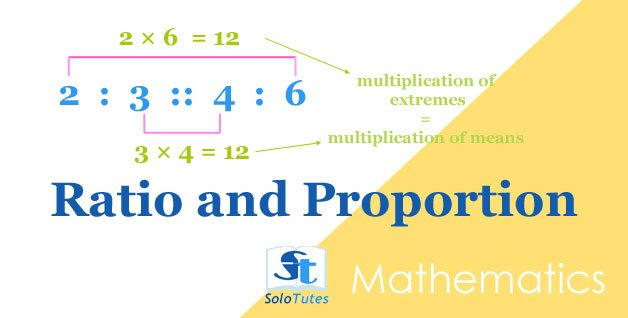SoloTutes

4 Posts

### All## Ratio and Proportion, Basic Tricks and Practice Problems## Ratio

1 of 3 〉 What number has 5 to 1 ratio to the number 10?
• 42
• 55
• 50
• 60
2 of 3 〉 There are 120 students in a picnic tour, if ratio of girls and boys is 5 : 7 then how many boys are in this tour?
• 50
• 70
• 55
• 120

1 more 👇## Practice Problems on Average | Math Quiz

1 of 3 〉 The average weight of A, B and C is  45 kg, that of A and B is 40 kg and B and C is 43 kg. What is the weight of B?
• 17 kg
• 26 kg
• 20 kg
• 31 kg
2 of 3 〉 The average of following 5 numbers is 8, find the missing number in 7, 5, 3,12,?.
• 14
• 13
• 8
• 12

1 more 👇

### Quiz / Practice Tests## Ratio

1 of 3 〉 What number has 5 to 1 ratio to the number 10?
• 42
• 55
• 50
• 60
2 of 3 〉 There are 120 students in a picnic tour, if ratio of girls and boys is 5 : 7 then how many boys are in this tour?
• 50
• 70
• 55
• 120

1 more 👇## Practice Problems on Average | Math Quiz

1 of 3 〉 The average weight of A, B and C is  45 kg, that of A and B is 40 kg and B and C is 43 kg. What is the weight of B?
• 17 kg
• 26 kg
• 20 kg
• 31 kg
2 of 3 〉 The average of following 5 numbers is 8, find the missing number in 7, 5, 3,12,?.
• 14
• 13
• 8
• 12

1 more 👇